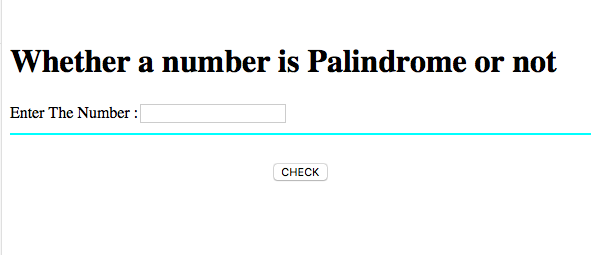# Check number is palindrome or not in JavaScript

Here, we are going to learn how to check whether a given number is palindrome or not in JavaScript.
Submitted by Aleesha Ali, on March 24, 2018

## Palindrome number

When a number doesn’t change it’ s value or meaning even after rotation from left to right or vice a versa, then the number is palindrome number.

For example: 12321 after rotation 12321number is still same.

### JavaScript code to check palindrome number

```<script>
function Palindrome()
{
var rem, temp, final = 0;
var number = Number(document.getElementById("N").value);

temp = number;
while(number>0)
{
rem = number%10;
number = parseInt(number/10);
final = final*10+rem;
}
if(final==temp)
{
}
else
{
window.alert("The inputted number is not palindrome");
}
}
</script>
```

Calling JavaScript function in HTML (HTML, JS Code)

```<html>
<script>
function Palindrome()
{
var rem, temp, final = 0;
var number = Number(document.getElementById("N").value);

temp = number;
while(number>0)
{
rem = number%10;
number = parseInt(number/10);
final = final*10+rem;
}
if(final==temp)
{
}
else
{
window.alert("The inputted number is not palindrome");
}
}
</script>
<body>
<br>
<h1>Whether a number is Palindrome or not</h1>
Enter The Number :<input type="text" name="n" id = "N"/>
<hr color="cyan">
<br>
<center><button onClick="Palindrome()">CHECK</button>
</body>
</html>
```

Output